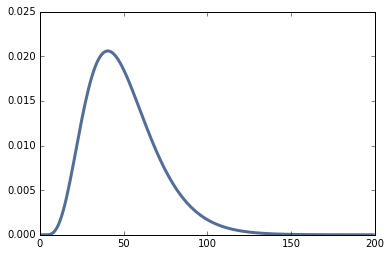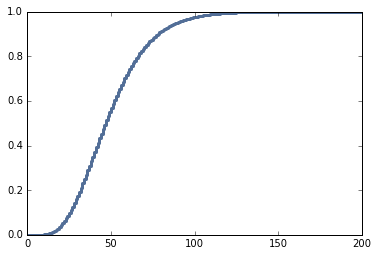## Wednesday, May 11, 2016

### Binomial and negative binomial distributions

Today's post is prompted by this question from Reddit:

How do I calculate the distribution of the number of selections (with replacement) I need to make before obtaining k? For example, let's say I am picking marbles from a bag with replacement. There is a 10% chance of green and 90% of black. I want k=5 green marbles. What is the distribution number of times I need to take a marble before getting 5?

I believe this is a geometric distribution. I see how to calculate the cumulative probability given n picks, but I would like to generalize it so that for any value of k (number of marbles I want), I can tell you the mean, 10% and 90% probability for the number of times I need to pick from it.

Another way of saying this is, how many times do I need to pull on a slot machine before it pays out given that each pull is independent?

Note: I've changed the notation in the question to be consistent with convention.

In :
from __future__ import print_function, division

import thinkplot
from thinkstats2 import Pmf, Cdf

from scipy import stats
from scipy import special

%matplotlib inline


### Solution¶

There are two ways to solve this problem. One is to relate the desired distribution to the binomial distribution.

If the probability of success on every trial is p, the probability of getting the kth success on the nth trial is

PMF(n; k, p) = BinomialPMF(k-1; n-1, p) p



That is, the probability of getting k-1 successes in n-1 trials, times the probability of getting the kth success on the nth trial.

Here's a function that computes it:

In :
def MakePmfUsingBinom(k, p, high=100):
pmf = Pmf()
for n in range(1, high):
pmf[n] = stats.binom.pmf(k-1, n-1, p) * p
return pmf


And here's an example using the parameters in the question.

In :
pmf = MakePmfUsingBinom(5, 0.1, 200)
thinkplot.Pdf(pmf)We can solve the same problem using the negative binomial distribution, but it requires some translation from the parameters of the problem to the conventional parameters of the binomial distribution.

The negative binomial PMF is the probability of getting r non-terminal events before the kth terminal event. (I am using "terminal event" instead of "success" and "non-terminal" event instead of "failure" because in the context of the negative binomial distribution, the use of "success" and "failure" is often reversed.)

If n is the total number of events, n = k + r, so

r = n - k



If the probability of a terminal event on every trial is p, the probability of getting the kth terminal event on the nth trial is

PMF(n; k, p) = NegativeBinomialPMF(n-k; k, p) p



That is, the probability of n-k non-terminal events on the way to getting the kth terminal event.

Here's a function that computes it:

In :
def MakePmfUsingNbinom(k, p, high=100):
pmf = Pmf()
for n in range(1, high):
r = n-k
pmf[n] = stats.nbinom.pmf(r, k, p)
return pmf


Here's the same example:

In :
pmf2 = MakePmfUsingNbinom(5, 0.1, 200)
thinkplot.Pdf(pmf2)And confirmation that the results are the same within floating point error.

In :
diffs = [abs(pmf[n] - pmf2[n]) for n in pmf]
max(diffs)

Out:
8.6736173798840355e-17

Using the PMF, we can compute the mean and standard deviation:

In :
pmf.Mean(), pmf.Std()

Out:
(49.998064403376738, 21.207570382894403)

To compute percentiles, we can convert to a CDF (which computes the cumulative sum of the PMF)

In :
cdf = Cdf(pmf)
scale = thinkplot.Cdf(cdf)And here are the 10th and 90th percentiles.

In :
cdf.Percentile(10), cdf.Percentile(90)

Out:
(26, 78)

Copyright 2016 Allen Downey

MIT License: http://opensource.org/licenses/MIT